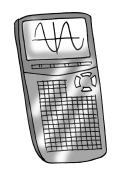### Home > CCA2 > Chapter Ch7 > Lesson 7.1.5 > Problem7-77

7-77.Your scientific or graphing calculator can function in both degrees and radians. See if you can determine how to put your calculator in radian mode and then how to switch it back to degree mode. On most scientific calculators, a small “DEG” or “RAD” shows on the screen to let you know in which mode you are working.

1. With your calculator in degree mode, find $\sin60^\circ$ and record your answer. Then switch to radian mode and find $\sin\frac{\pi}{3}$. Did you get the same answer? Explain why your answers should be the same or different.

What is $60^\circ$  in radians?  $\frac{\pi}{3}$ in degrees?

2. Find $\sin\frac{\pi}{4}$. Which angles, measured in degrees, would have the same sine as $\sin\frac{\pi}{4}$?

$45^\circ, 135^\circ, 405^\circ, \text{etc.}$